#Function Repository Resource:

# Tetartoid

Get a tetartoid given three values

Contributed by: Ed Pegg Jr (Wolfram Research)
 ResourceFunction["Tetartoid"][{a,b,c}] returns a tetartoid, given the non-negative values a,b and c. ResourceFunction["Tetartoid"][{a,b,c},"Polyhedron"] returns a tetartoid represented as a Polyhedron object.

## Details

A tetartoid is a dodecahedron with 12 identical irregular pentagonal faces and that has chiral tetrahedral symmetry (point group T in Schönflies notation).
In cases of degeneracy, a default tetartoid will be returned instead.

## Examples

### Basic Examples (1)

A sample tetartoid:

 In:=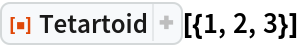Out=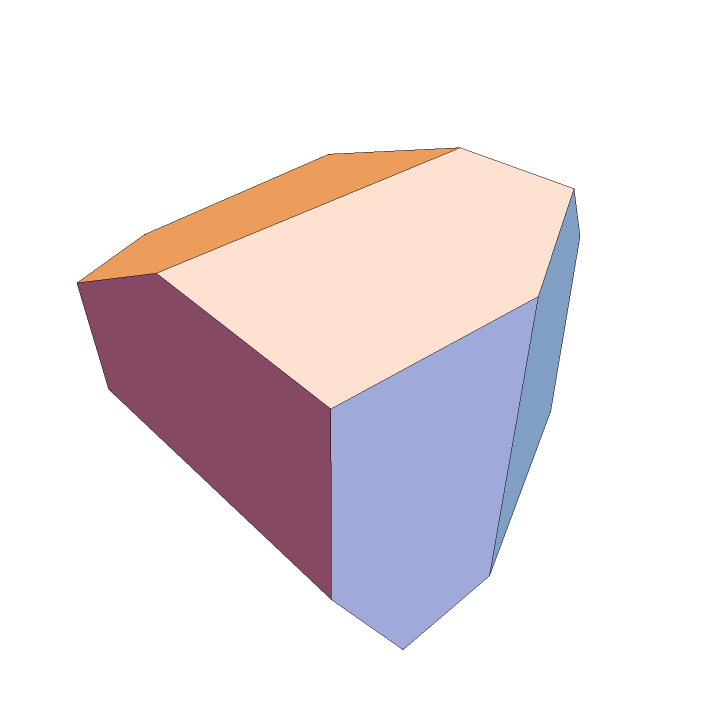### Scope (4)

Return a tetartoid as a Polyhedron object:

 In:=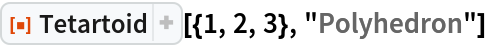Out=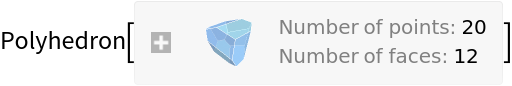In:=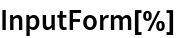Out=Compute its surface area and volume:

 In:=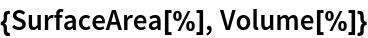Out=The tetartoid generally has chiral tetrahedral symmetry:

 In:=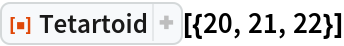Out=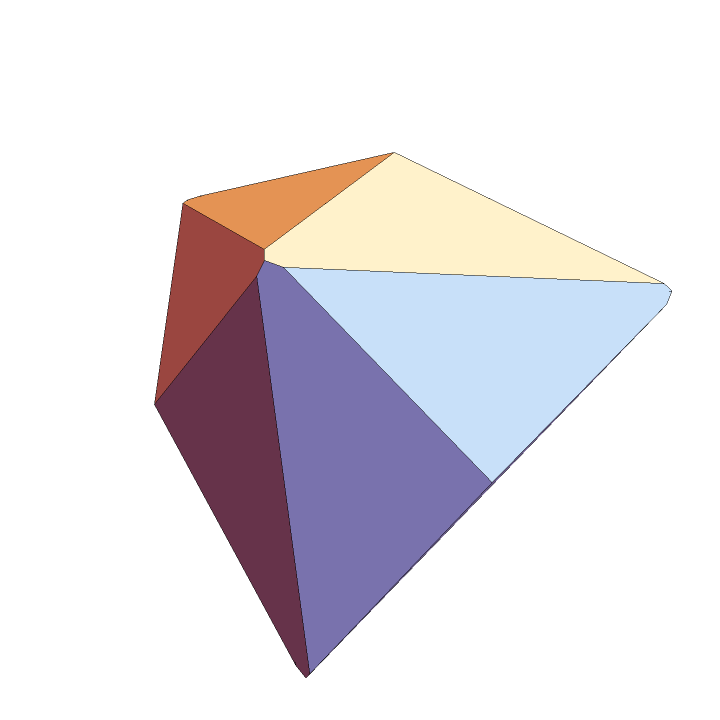The tetartoid can have dodecahedral symmetry:

 In:=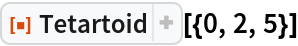Out=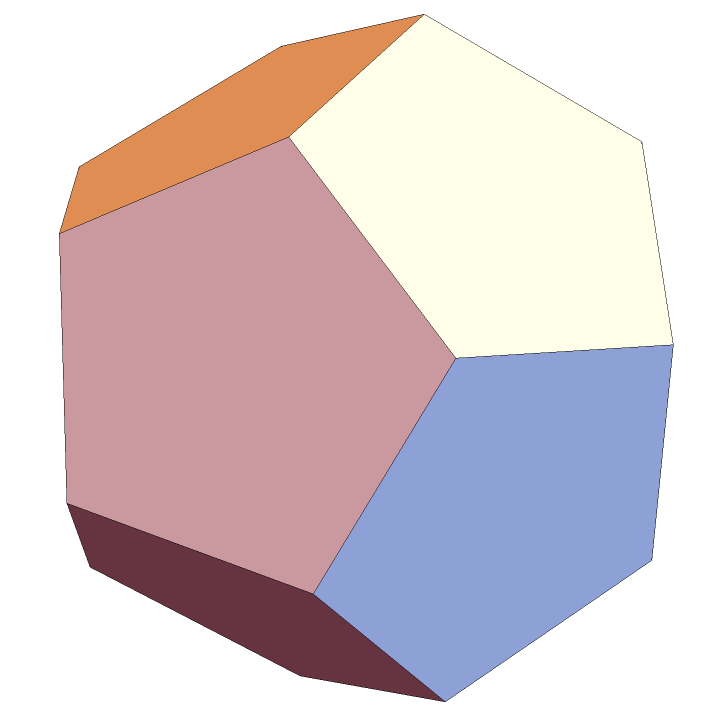The tetartoid can have cubic symmetry:

 In:=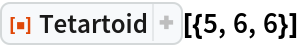Out=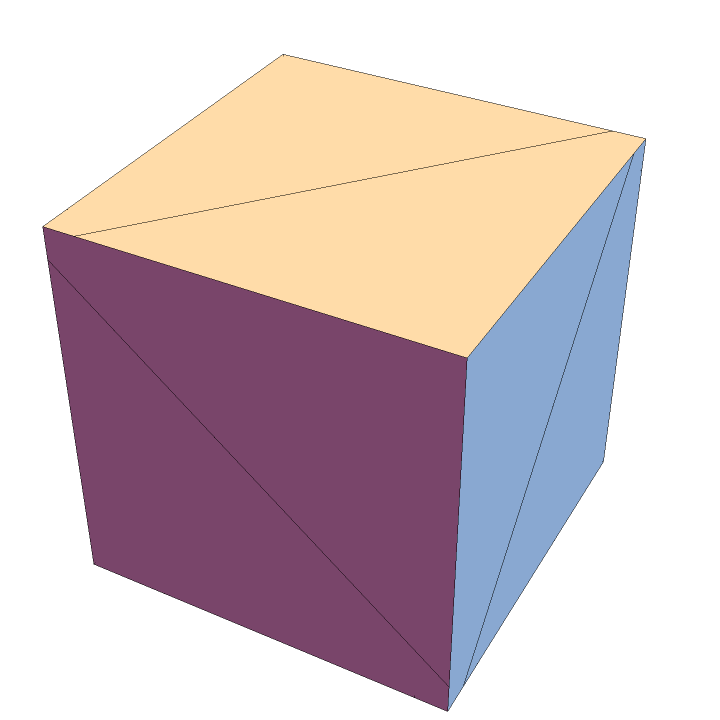### Possible Issues (1)

The construction calculates some denominators that cannot be zero. In cases where a zero is encountered, a default tetartoid is substituted: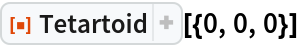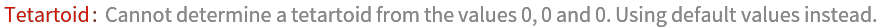Out=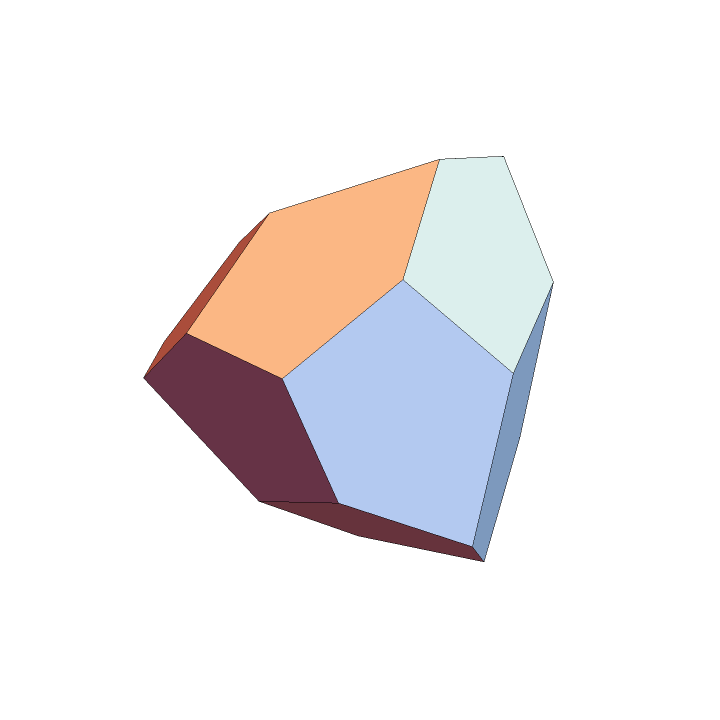## Requirements

Wolfram Language 11.3 (March 2018) or above